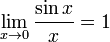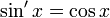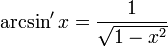In mathematics, a radian is the angle subtended by an arc length equal to the radius of a circle. There are 2π radians in a full circle, as the arc length of the circumference of a circle is equal to 2π times the radius of the circle.

The radian is considered a dimensionless derived unit in the International System of Units.

Measuring angles in radians often allows the direct use of angles in mathematical formulae, particularly in trigonometric functions and their expansions.

Generally, identities arising in calculus and involving trigonometric functions are true only when the variables that are arguments to trigonometric functions are expressed in radians. Among those are the following:$\lim_{x\to 0}\frac{\sin x}{x} = 1$$\sin' x = \cos x \,$$\arcsin' x = \frac{1}{\sqrt{1 - x^2}}$

## Other units

• 1 radian is equal to 180 / π or 57.295 779 513... degrees of arc
• 1 radian is equal to 200 / π or 63.661 977 237... grads

## Trigonometric functions

• The sine of 1 radian is 0.841 470 985...
• The cosine of 1 radian is 0.540 302 306...
• The tangent of 1 radian is 1.557 407 775...Some content on this page may previously have appeared on Citizendium.